Courses

# Ex 14.4 NCERT Solutions- Statistics Class 9 Notes | EduRev

## Class 9 Mathematics by Full Circle

Created by: Full Circle

## Class 9 : Ex 14.4 NCERT Solutions- Statistics Class 9 Notes | EduRev

The document Ex 14.4 NCERT Solutions- Statistics Class 9 Notes | EduRev is a part of the Class 9 Course Class 9 Mathematics by Full Circle.
All you need of Class 9 at this link: Class 9

Question 1. The following number of goals were scored by a team in a series of 10 matches:
2, 3, 4, 5, 0, 1, 3, 3, 4, 3
Find the mean, median and mode of these scores.
Solution:
To find mean: Here, n = 10,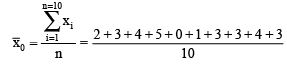= (28/10) = 2.8
∴ Mean = 2.8
To find median:
Arranging the given data in an ascending order, we have: 0, 1, 2, 3, 3, 3, 3, 4, 4, 5
For even observation, we have median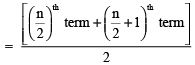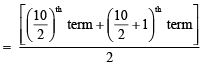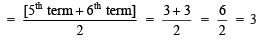Thus, the median of the data = 3
To find the mode:
∵ In the given data 0 occurs 1 time, 1 occurs 1 time, 2 occurs 1 time, 3 occurs 4 times, 4 occurs 2 times, 5 occurs 1 time
∵ 3 occurs maximum number of times.
∴ Mode = 3.

Question 2. In a Mathematics test given to 15 students, the following marks (out of 100) are recorded:
41, 39, 48, 52, 46, 62, 54, 40, 96, 52, 98, 40, 42, 52, 60
Find the mean, median and mode of this data.
Solution:
To find the mean: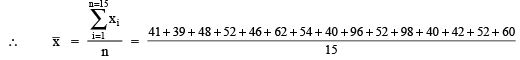=(822/15) = 54.8
Thus, mean = 54.8
To find median:
Arranging the given data in an ascending order, we have:
39, 40, 40, 41, 42, 46, 48, 52, 52, 52, 54, 60, 62, 96, 98
∵ n = 15, an odd number

∴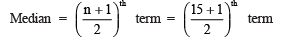= (16/2)th term = 8th term = 52

Thus, the median = 52
To find mode:
∵ In the given data, the observation 52 occurs 3 times, i.e., the maximum number of times.
∴ Mode = 52

Question 3. The following observations have been arranged in ascending order. If the median of the data is 63, find the value of x.
29, 32, 48, 50, x, x+2, 72, 78, 84, 95
Solution:
Here, the given observations are in an ascending order.
∵ n = 10 (an even number of observations)

∴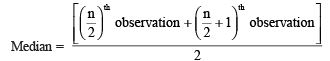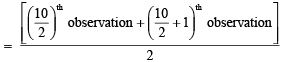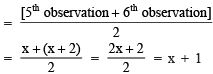Since, median = 63                   [Given]
∴ x + 1 = 63
⇒   x = 63 – 1 = 62
Thus, the required value of x is 62.

Question 4. Find the mode of 14, 25, 14, 28, 18, 17, 18, 14, 23, 22, 14, 18.
Solution:
Arranging the given data in an ascending order: 14, 14, 14, 14, 17, 18, 18, 18, 22, 23, 25, 28.
∴ The observation 14 is occurring the maximum number of times (i.e., 4 times)
∴ Mode of the given data = 14

Question 5. Find the mean salary of 60 workers of factory from the following table:

 Salary in ₹ Number of workers 3000 16 4000 12 5000 10 6000 8 7000 6 8000 4 9000 3 10000 1 Total 60

Solution: Let salaries be represented by xi and number of corresponding workers by fi.
Note: The mean x of n observations having values as x1, x2, x3, …, xand occurring with frequencies f1, f2, f3, …, fn respectively is given by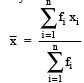Here,
fx x1 = 16 x 3000 = 48,000
f2 x x2 = 12 x 4000 = 48,000
f3 x x3 = 10 x 5000 = 50,000
fx x4 = 8 x 6000 = 48,000
f5 x x5 = 6 x 7000 = 42,000
f6 x x= 4 x 8000 = 32,000
f7 x x= 3 x 9000 = 27,000
f8 x x8 = 1 x 10000 = 10,000

∴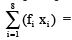= [48000 + 48000 + 50000 + 48000 + 42000 + 32000 + 27000 + 10000]
= 305000

and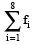= 16 + 12 + 10 + 8 + 6 + 4 + 3 + 1 = 60

⇒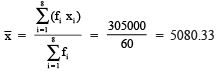Thus, the mean salary = ₹ 5080.33

 salary in ₹ (xi) number of workers (fi) (fi xi) 3000 16 16 x 3000 = 48000 4000 12 12 x 4000 = 48000 5000 10 10 x 5000 = 50000 6000 8 8 x 6000 = 48000 7000 6 6 x 7000 = 42000 8000 4 4 x 8000 = 32000 9000 3 3 x 9000 = 27000 10000 1 1 x 10000 = 10000 Total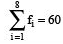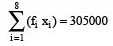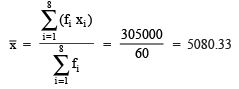Thus, the required mean salary = ₹ 5080.33

Question 6. Give one example of a situation in which
(i) The mean is an appropriate measure of central tendency.
(ii) The mean is not an appropriate measure of central tendency but the median is an appropriate measure of central tendency.

Solution: (i) Mean is a quantitative central tendency of a data.
Example: For measuring central tendency of marks of a test we find the mean of the data.
(ii) Median is a qualitative central tendency of a data.
Example: For measuring central tendency of beauty of a group of women, we determine the median of the data.

,

,

,

,

,

,

,

,

,

,

,

,

,

,

,

,

,

,

,

,

,

;